# Sales Forecast Example - Models comparison and selection

This paper will apply econometric techniques to build a 6-month forecast for a sales force at company X. We will use the monthly total sales figures for the last 25 months for sample data.

Our objective is to compare competing models and to define a guideline for selecting the best model.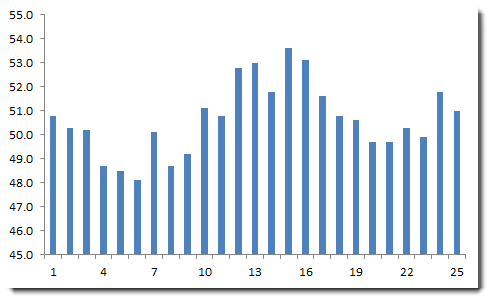## Step 1: Summary Statistics

Using the Descriptive Statistic wizard (pictured below), examine the different summary stats for the sample data.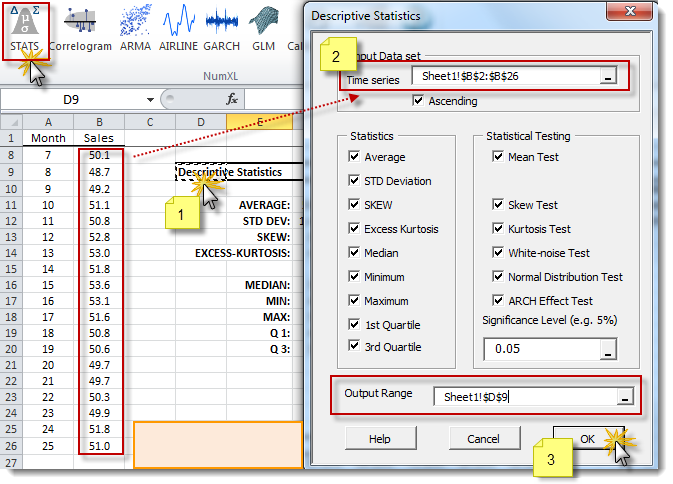In the summary statistics table (pictured below), the data series exhibits serial correlation (i.e. failed the white noise test) and fat tails (significant excess kurtosis and ARCH effect).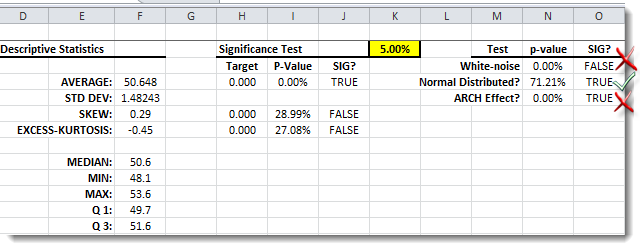## Step 2: Correlogram Analysis

Using the NumXL toolbar, launch the Correlogram wizard.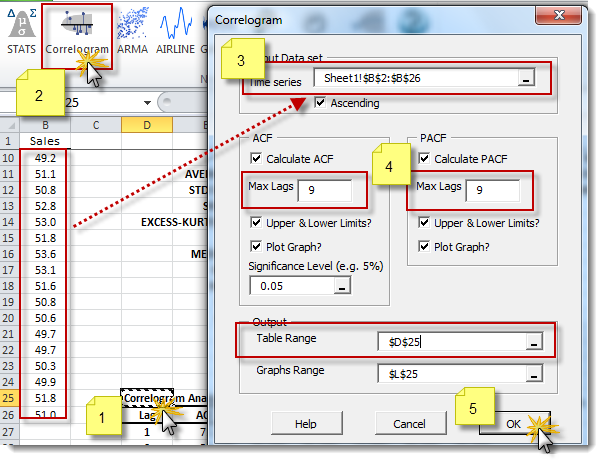Highlight the log data and select 9 lags for ACF and PACF. Then, create a correlogram for the data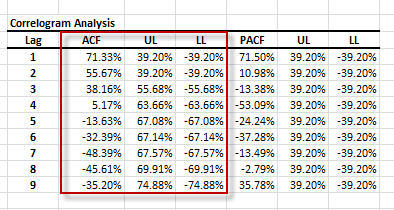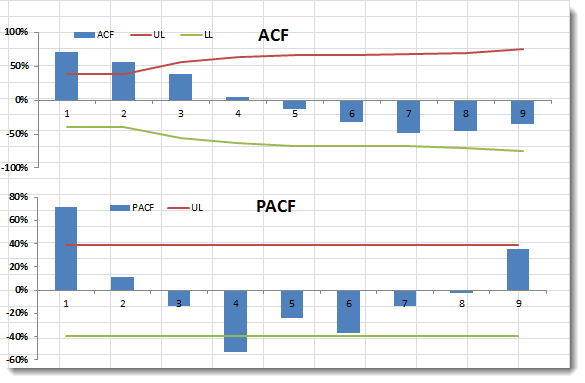Examining the ACF and PACF plots, the data appear to be driven from an ARMA process with an AR order of One(1) and an MA order of two(2). The data sample is relatively small, so be careful not to over-fit the data with a high-order model.

## Step 3: ARMA Modeling

Based on the ACF/PACF plot, we can propose an ARMA model for our data. Furthermore, the AR or the MA order is less than or equal to two (2).

$$\left(1-\sum_{i=1}^p{\phi_i L^i}\right)\left(x_t-\mu\right)=\left(1+\sum_{j=1}^q{\theta_j L^j}\right)a_t$$

Where:

• $L$ = the lag or backshift operator
• $\phi_i$ = the i-th coefficient of the AR component.
• $p$ = the order of the autoregressive (AR) component.
• $x_t$ = the monthly total sales at month $t$
• $\mu$ = the long-run mean of the the ARMA process
• $\theta_j$ = the j-th coefficient of the MA component
• $q$ = the order of the moving average (MA) component
• $a_t$ = the residual, shock, innovation or error term at month $t$.

In this section, we’ll use a brute force approach and examine all possible ARMA model permutations. In sum, we will specify, calibrate, validate and, finally, compare each model against the others to determine the best (and simplest) fit.

### Step 3.1: Model Specification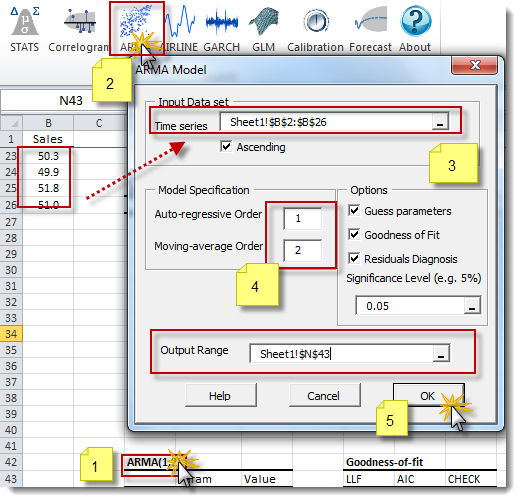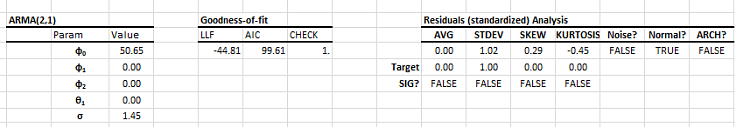### Step 3.2: Model Calibration

Select the cell at the top of the airline model table (i.e. “ARMA(1,2)”) and click on the Calibration icon in the toolbar.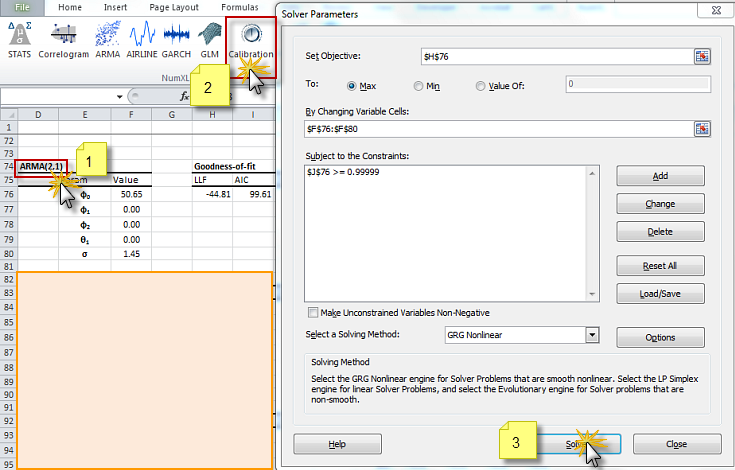### Step 3.3: Examine and Validate the Calibrated Model

For the ARMA(2.1) model above, the new optimal values for the model parameters are shown below: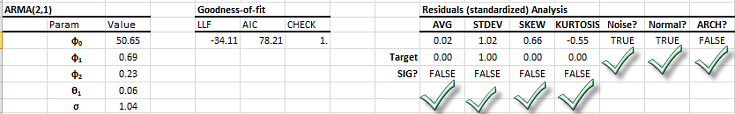### Step 3.4: Compare the Models and Select the Best One

In the attached spreadsheet, we repeated the previous step (3.1-3.3) for all ARMA models of order (1,0) to (2,2). The following table summarizes our findings: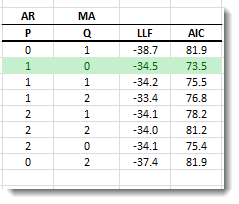The calculation of Akaike’s information criterion (AIC) takes into account the complexity of the model, and it penalizes the fit as the number of free arguments increases. For comparing different models, we will use the AIC measure.

Although the AICs for all the models are comparably close, we favor the simplest method and chose ARMA(1,0) or AR(1).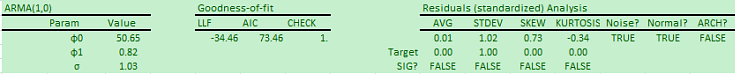## Step 4: Forecast

The residuals of the selected calibrated model satisfy the assumptions of the ARMA model. Now, we are ready to conduct a 6-month forecast for the monthly total sales.

Select the cell labeled “ARMA(1,0)” and click on the Forecast icon in the toolbar.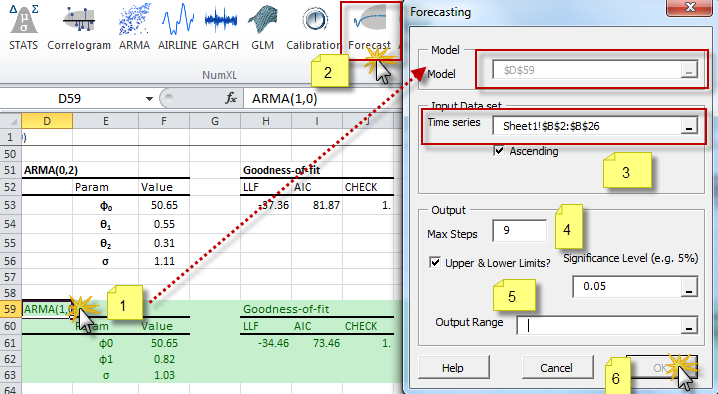Please note that for forecast purposes, the input time series refers to the latest sales total figures (i.e. last month). The output table is shown below: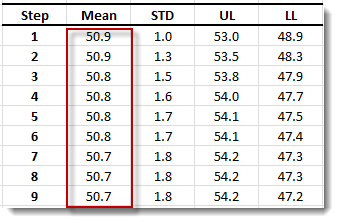Please note: the forecast values are drifting over time toward the model’s long-run mean of 50.65 ($\phi_o$ ), and the forecast errors are approaching 1.8; the model’s marginal (i.e. unconditional) long-run standard deviation.

Finally, plot the forecast mean and the confidence interval for the next 9-12 months, as shown below: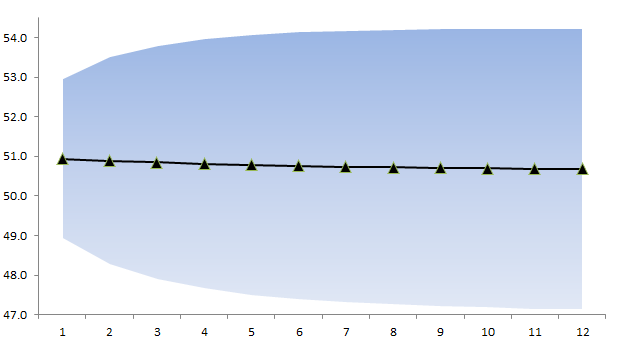## Conclusion

Now, we selected the ARMA(1,0) or AR(1) model as the best fit for the sample data, we examine the practical implication of its representation. The model indicates a significant correlation for sales figures between consecutive months.

Furthermore, about forty-five percent (45%) of the sales-cases (that company X won), are closed in two month cycle:

$$r = \frac{\phi_1}{1+\phi_1}=\frac{0.82}{1.82}=45\%$$